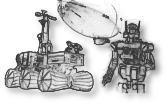## math/ros-geometry

ROS geometry library (including TF frame management)
Directory:
math/ros-geometry (package's history)
Package version:
ros-geometry-1.12.0r1
http://ros.org/wiki/geometry
modified-bsd
Source archive:
https://github.com/ros/geometry/archive/1.12.0.tar.gz
Description:
The ros-geometry package contains several tools to deal with manipulation of geometry entities like frames. - tf tf is a package that lets the user keep track of multiple coordinate frames over time. tf maintains the relationship between coordinate frames in a tree structure buffered in time, and lets the user transform points, vectors, etc between any two coordinate frames at any desired point in time. - {tf, kdl, eigen}_conversions functions to convert between TF, KDL, Eigen and ROS datatypes
Run dependencies:
googletest, libstdc++, orocos-kdl>=1.1, py27-catkin-pkg>=0.2, py27-empy>=3, py27-nose>=0.10, py27-pyparsing>=1, ros-actionlib>=1.11<1.13, ros-angles>=1.9, py27-ros-catkin>=0.7<0.8, ros-comm>=1.13<1.15, ros-common-msgs>=1.12<1.13, ros-genmsg>=0.5<0.7, ros-genpy>=0.6<0.7, ros-geometry2>=0.6<0.7, ros-message-generation>=0.4<0.5, ros-std-msgs>=0.5
Build dependencies:
boost-headers>=1.60, cmake>=2.8.3, digest>=20080510, eigen3>=3.0.0, g++>=3, gcc>=3, patch>=2.0, pax, pkg-config>=0.22, pkg_install>=20110805.12, python27>=2.7<2.8, ros-cmake-modules>=0.3, tnftp>=20130505~ssl
General options:
debug
Produce debugging information for binary programs
c++-compiler alternatives:
g++
Use the GNU C++ compiler
clang++
Use the LLVM C++ compiler
ccache-g++
Use ccache and the GNU C++ compiler
ccache-clang++
Use ccache and the LLVM C++ compiler
c-compiler alternatives:
gcc
Use the GNU C compiler
clang
Use the LLVM C compiler
ccache-gcc
Use ccache and the GNU C compiler
ccache-clang
Use ccache and the LLVM C compiler
python alternatives:
python27
Use python-2.7
python36
Use python-3.6
python37
Use python-3.7
python38
Use python-3.8
python39
Use python-3.9
python310
Use python-3.10
python311
Use python-3.11
ros alternatives:
melodic
Use ros melodic